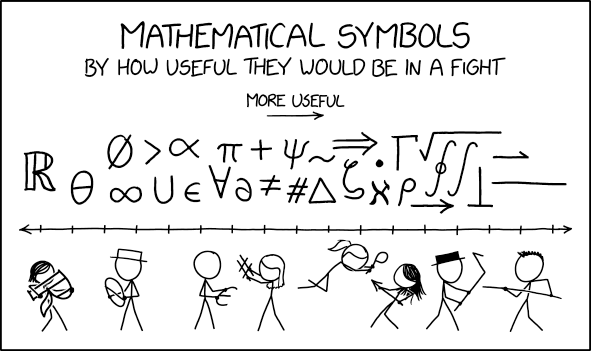# My Favorite One-Liners: Part 122

Once in my probability class, a student asked a reasonable question — could I intuitively explain the difference between “uncorrelated” and “independent”? This is a very subtle question, as there are non-intuitive examples of random variables that are uncorrelated but are nevertheless dependent. For example, if$X$ is a random variable uniformly distributed on$\{-1,0,1\}$ and$Y= X^2$, then it’s straightforward to show that$E(X) = 0$ and$E(XY) = E(X^3) = E(X) = 0$, so that$\hbox{Cov}(X,Y) = E(XY) - E(X) E(Y) = 0$

and hence$X$ and$Y$ are uncorrelated.

However, in most practical examples that come up in real life, “uncorrelated” and “independent” are synonymous, including the important special case of a bivariate normal distribution.

This was my expert answer to my student: it’s like the difference between “mostly dead” and “all dead.”

# My Favorite One-Liners: Part 121

I’ll use this one-liner when I ask my students to do something that’s a little conventional but nevertheless within their grasp. For example, consider the following calculation using a half-angle trigonometric identity:$\cos \displaystyle \frac{5\pi}{8} = \cos \displaystyle \left( \frac{1}{2} \cdot \frac{5\pi}{4} \right)$$= \displaystyle - \sqrt{ \frac{1 + \cos 5\pi/4}{2} }$$= \displaystyle - \sqrt{ \frac{ 1 - \displaystyle \frac{\sqrt{2}}{2}}{2} }$$= \displaystyle - \sqrt{ \frac{ ~~~ \displaystyle \frac{2-\sqrt{2}}{2} ~~~}{2} }$$= \displaystyle - \sqrt{ \frac{2 - \sqrt{2}}{4}}$$= \displaystyle - \frac{ \sqrt{2 - \sqrt{2}}}{\sqrt{4}}$$= \displaystyle - \frac{ \sqrt{2 - \sqrt{2}}}{2}$

That’s certainly a very complicated calculation, with plenty of predictable places where a student might make an inadvertent mistake.

In my experience, one somewhat surprising place that can trip up students seeing such a calculation for the first time is the very first step: changing$\displaystyle \frac{5\pi}{8}$ into$\displaystyle \frac{1}{2} \cdot \frac{5\pi}{4}$. Upon reflection, perhaps this isn’t so surprising: students are very accustomed to taking a complicated expression like$\displaystyle \frac{1}{2} \cdot \frac{5\pi}{4}$ and making it simpler. However, they aren’t often asked to take a simple expression like$\displaystyle \frac{5\pi}{8}$ and make it more complicated.

So I try to make this explicitly clear to my students. A lot of times, we want to make a complicated expression simple. Sometimes, we have to go the other direction and make a simple expression more complicated. Students should be able to do both. And, to try to make this memorable for my students, I use my one-liner:

“In the words of the great philosopher, you gotta know when to hold ’em and know when to fold ’em.”

Yes, that’s an old song reference. My experience is that most students have heard the line before but unfortunately can’t identify the singer: the late, great Kenny Rogers.

# A line joining two infinitely small points

Been there, done that.# Usefulness of Mathematical Symbols in a FightSource: https://xkcd.com/2343/

# My Favorite One-Liners: Part 120

I used these shirts as props when teaching Precalculus this week, and they worked like a charm.After deriving the three Pythagorean identities from trigonometry, I told my class that I got these hand-made his-and-hers T-shirts for my wife’s birthday a couple of years ago. If you can’t see from the picture, one says$\sin^2 \theta$ and the other$\cos^2 \theta$.

After holding up the shirts, I then asked the class what mathematical message was being communicated.

After a few seconds, someone ventured a guess: “We add up to 1?”

I answered, “That’s right. Together, we’re one.”

Whereupon the class spontaneously reacted with a loud “Awwwwwwwwww.”

I was exceedingly happy.

# Fun with dimensional analysisSource: https://xkcd.com/2327/